# Leibnitz Theorem

Leibnitz Theorem is basically the Leibnitz rule defined for derivative of the antiderivative. As per the rule, the derivative on nth order of the product of two functions can be expressed with the help of a formula. The functions that could probably have given function as a derivative are known as antiderivatives (or primitive) of the function. The formula that gives all these antiderivatives is called the indefinite integral of the function, and such process of finding antiderivatives is called integration. Now let us discuss here the formula and proof of Leibnitz rule.

## Leibnitz Theorem Formula

Suppose there are two functions u(t) and v(t), which have the derivatives up to nth order. Let us consider now the derivative of the product of these two functions.

The first derivative could be written as;

(uv)’ = u’v+uv’

Now if we differentiate the above expression again, we get the second derivative;

(uv)’’

= [(uv)’]’

= (u’v+uv’)’

= (u’v)’+(uv’)’

= u′′v + u′v′ + u′ v′ + uv′′

= u′′v + 2u′v′ + uv′′

Similarly, we can find the third derivative;

(uv)′′′

= [(uv)′′]′

= (u′′v + 2u′v′ + uv′′)′

= (u′′v)′ + (2u′v′)′ + (uv′′)′

= u′′′v + u′′v′ + 2u′′v′ + 2u′v′′ + u′v′′ + uv′′′

= u′′′v + 3u′′v′ + 3u′v′′ + uv′′′

Now if we compare these expressions, it is found very similar to binomial expansion raised to the exponent. If we consider the terms with zero exponents, u0 and v0 which correspond to the functions u and v themselves, we can generate the formula for nth order of the derivative product of two functions, in a such a way that;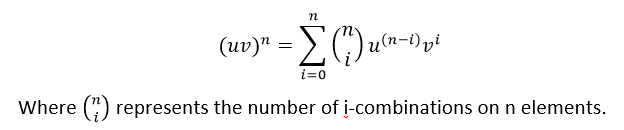This formula is known as Leibniz Rule formula and can be proved by induction.

## Leibnitz Theorem Proof

Assume that the functions u(t) and v(t) have derivatives of (n+1)th order. By recurrence relation, we can express the derivative of (n+1)th order in the following manner: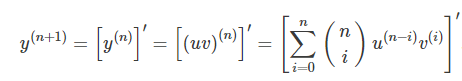Upon differentiating we get;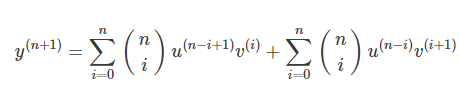The summation on the right side can be combined together to form a single sum, as the limits for both the sum are the same. Now, let us take an intermediate index such that 1≤m≤n. So, when i = m, then the first term can be written as;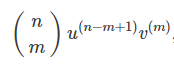The second term when i=m-1 will be;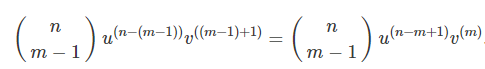On adding these two terms, we get;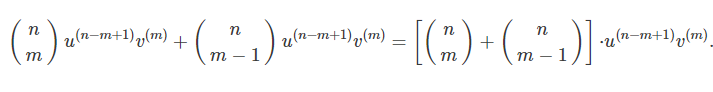We know from the concept of combinatorics that;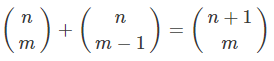Based on the above concept, we can write the sum of these two terms, when i =m and when i = m-1, as;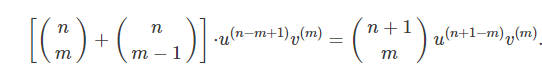From the above expression, we can see when the value of m changes from 1 to n, this generated combination will cover all the terms from i= 1 to i=n, but not i=0 in the first term and i = 1 in the second term which are equal to;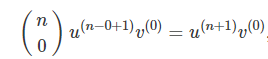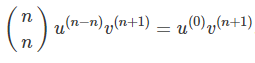Hence, the resulted derivative of (n+1)th order of the product of two functions is given by;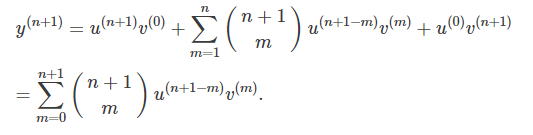Hence, we have derived here the Leibnitz formula.